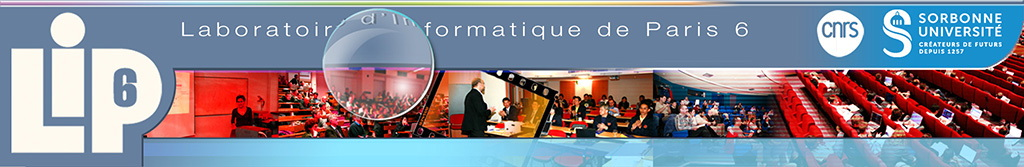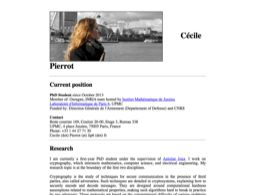﻿ PIERROT Cécile# PIERROT Cécile

Tiến sĩ
Nhóm nghiên cứu : ALMASTY
Ngày đi : 31-12-2016
Ban lãnh đạo nghiên cứu : Antoine JOUX

#Discrete logarithm in finite fields

Cryptography is the study of techniques for secure communication in the presence of third parties, also called adversaries. Such techniques are designed around computational hardness assumptions related to mathematical properties, making such algorithms hard to break in practice by any adversary. These protocols are based on the computational difficulty of various problems which often come from number theory, such as integer factorization or discrete logarithms computations.
This thesis focuses on the discrete logarithm problem in the multiplicative group of invertible elements of a finite field.
First we propose a quasipolynomial time algorithm build upon Frobenius representation of the target field that permits to solve the problem if the characteristic is small, with respect to the whole order of the field. In practice and for instance, the current discrete logarithm record in characteristic 3 uses this result.
For medium or large characteristics finite fields, another approach is required. So we present the multiple number field sieve (MNFS) that reaches the best asymptotic heuristic complexities for this double range of characteristics. Last but not least, we also introduce the notion of nearly sparse matrices. Designing a new algorithm dedicated to explicitly give the kernel of such a matrix eases in practice the linear algebra step of any variant of the number field sieve.
Bảo vệ luận án : 25-11-2016 - 14h30 - Campus Jussieu- Tour Zamansky Salle 24è étage
Hội đồng giám khảo :
Reynald Lercier (Université Rennes 1) [rapporteur]
Daniel Augot (Inria, Ecole Polytechnique)
Ronald Cramer (CWI, Amsterdam)
Guillaume Hanrot (ENS Lyon)
Antoine Joux (Fondation partenariale de l'UPMC)
Ariane Mézard (UPMC)
David Naccache (ENS Paris)